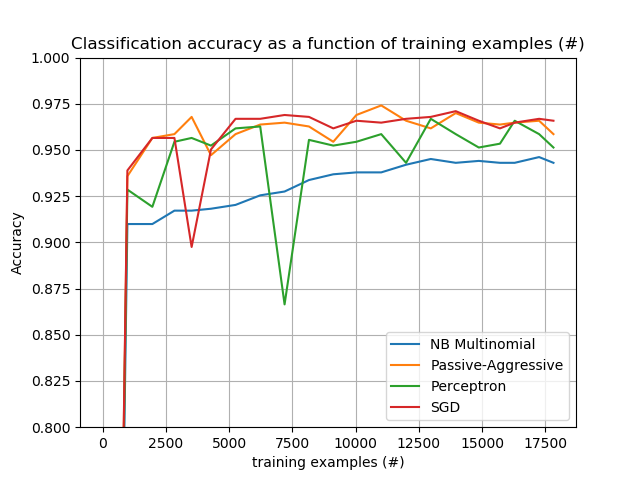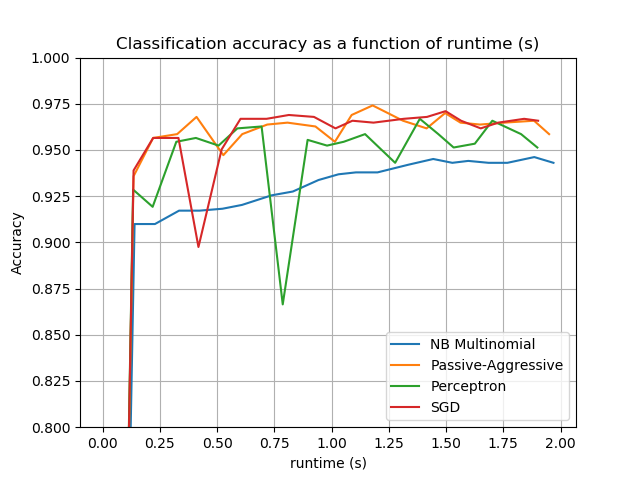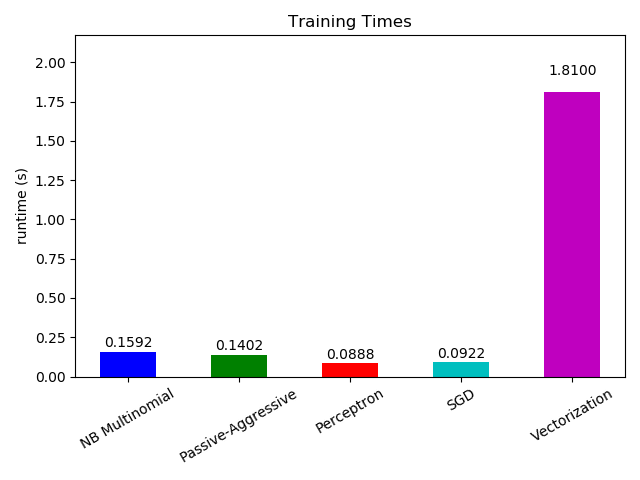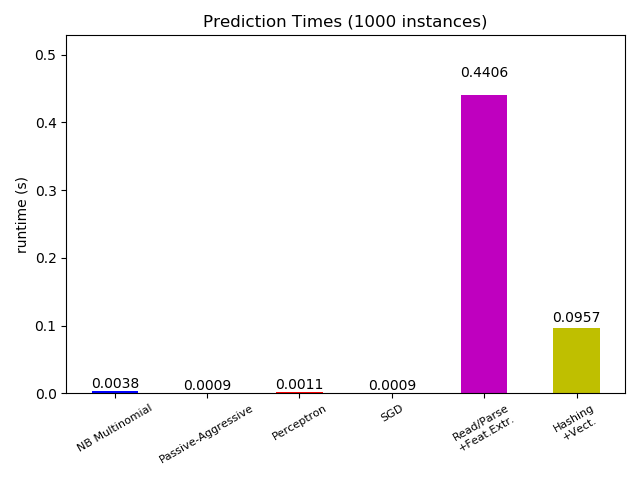# Out-of-core classification of text documents¶

This is an example showing how scikit-learn can be used for classification using an out-of-core approach: learning from data that doesn’t fit into main memory. We make use of an online classifier, i.e., one that supports the partial_fit method, that will be fed with batches of examples. To guarantee that the features space remains the same over time we leverage a HashingVectorizer that will project each example into the same feature space. This is especially useful in the case of text classification where new features (words) may appear in each batch.

The dataset used in this example is Reuters-21578 as provided by the UCI ML repository. It will be automatically downloaded and uncompressed on first run.

The plot represents the learning curve of the classifier: the evolution of classification accuracy over the course of the mini-batches. Accuracy is measured on the first 1000 samples, held out as a validation set.

To limit the memory consumption, we queue examples up to a fixed amount before feeding them to the learner.

# Authors: Eustache Diemert <eustache@diemert.fr>
#          @FedericoV <https://github.com/FedericoV/>

from __future__ import print_function
from glob import glob
import itertools
import os.path
import re
import tarfile
import time
import sys

import numpy as np
import matplotlib.pyplot as plt
from matplotlib import rcParams

from sklearn.externals.six.moves import html_parser
from sklearn.externals.six.moves.urllib.request import urlretrieve
from sklearn.datasets import get_data_home
from sklearn.feature_extraction.text import HashingVectorizer
from sklearn.linear_model import SGDClassifier
from sklearn.linear_model import PassiveAggressiveClassifier
from sklearn.linear_model import Perceptron
from sklearn.naive_bayes import MultinomialNB

def _not_in_sphinx():
# Hack to detect whether we are running by the sphinx builder
return '__file__' in globals()


## Main¶

Create the vectorizer and limit the number of features to a reasonable maximum

vectorizer = HashingVectorizer(decode_error='ignore', n_features=2 ** 18,
alternate_sign=False)

# Iterator over parsed Reuters SGML files.
data_stream = stream_reuters_documents()

# We learn a binary classification between the "acq" class and all the others.
# "acq" was chosen as it is more or less evenly distributed in the Reuters
# files. For other datasets, one should take care of creating a test set with
# a realistic portion of positive instances.
all_classes = np.array([0, 1])
positive_class = 'acq'

# Here are some classifiers that support the partial_fit method
partial_fit_classifiers = {
'SGD': SGDClassifier(max_iter=5),
'Perceptron': Perceptron(tol=1e-3),
'NB Multinomial': MultinomialNB(alpha=0.01),
'Passive-Aggressive': PassiveAggressiveClassifier(tol=1e-3),
}

def get_minibatch(doc_iter, size, pos_class=positive_class):
"""Extract a minibatch of examples, return a tuple X_text, y.

Note: size is before excluding invalid docs with no topics assigned.

"""
data = [(u'{title}\n\n{body}'.format(**doc), pos_class in doc['topics'])
for doc in itertools.islice(doc_iter, size)
if doc['topics']]
if not len(data):
return np.asarray([], dtype=int), np.asarray([], dtype=int)
X_text, y = zip(*data)
return X_text, np.asarray(y, dtype=int)

def iter_minibatches(doc_iter, minibatch_size):
"""Generator of minibatches."""
X_text, y = get_minibatch(doc_iter, minibatch_size)
while len(X_text):
yield X_text, y
X_text, y = get_minibatch(doc_iter, minibatch_size)

# test data statistics
test_stats = {'n_test': 0, 'n_test_pos': 0}

# First we hold out a number of examples to estimate accuracy
n_test_documents = 1000
tick = time.time()
X_test_text, y_test = get_minibatch(data_stream, 1000)
parsing_time = time.time() - tick
tick = time.time()
X_test = vectorizer.transform(X_test_text)
vectorizing_time = time.time() - tick
test_stats['n_test'] += len(y_test)
test_stats['n_test_pos'] += sum(y_test)
print("Test set is %d documents (%d positive)" % (len(y_test), sum(y_test)))

def progress(cls_name, stats):
"""Report progress information, return a string."""
duration = time.time() - stats['t0']
s = "%20s classifier : \t" % cls_name
s += "%(n_train)6d train docs (%(n_train_pos)6d positive) " % stats
s += "%(n_test)6d test docs (%(n_test_pos)6d positive) " % test_stats
s += "accuracy: %(accuracy).3f " % stats
s += "in %.2fs (%5d docs/s)" % (duration, stats['n_train'] / duration)
return s

cls_stats = {}

for cls_name in partial_fit_classifiers:
stats = {'n_train': 0, 'n_train_pos': 0,
'accuracy': 0.0, 'accuracy_history': [(0, 0)], 't0': time.time(),
'runtime_history': [(0, 0)], 'total_fit_time': 0.0}
cls_stats[cls_name] = stats

get_minibatch(data_stream, n_test_documents)

# We will feed the classifier with mini-batches of 1000 documents; this means
# we have at most 1000 docs in memory at any time.  The smaller the document
# batch, the bigger the relative overhead of the partial fit methods.
minibatch_size = 1000

# Create the data_stream that parses Reuters SGML files and iterates on
# documents as a stream.
minibatch_iterators = iter_minibatches(data_stream, minibatch_size)
total_vect_time = 0.0

# Main loop : iterate on mini-batches of examples
for i, (X_train_text, y_train) in enumerate(minibatch_iterators):

tick = time.time()
X_train = vectorizer.transform(X_train_text)
total_vect_time += time.time() - tick

for cls_name, cls in partial_fit_classifiers.items():
tick = time.time()
# update estimator with examples in the current mini-batch
cls.partial_fit(X_train, y_train, classes=all_classes)

# accumulate test accuracy stats
cls_stats[cls_name]['total_fit_time'] += time.time() - tick
cls_stats[cls_name]['n_train'] += X_train.shape
cls_stats[cls_name]['n_train_pos'] += sum(y_train)
tick = time.time()
cls_stats[cls_name]['accuracy'] = cls.score(X_test, y_test)
cls_stats[cls_name]['prediction_time'] = time.time() - tick
acc_history = (cls_stats[cls_name]['accuracy'],
cls_stats[cls_name]['n_train'])
cls_stats[cls_name]['accuracy_history'].append(acc_history)
run_history = (cls_stats[cls_name]['accuracy'],
total_vect_time + cls_stats[cls_name]['total_fit_time'])
cls_stats[cls_name]['runtime_history'].append(run_history)

if i % 3 == 0:
print(progress(cls_name, cls_stats[cls_name]))
if i % 3 == 0:
print('\n')


Out:

Test set is 966 documents (88 positive)
/home/circleci/project/sklearn/linear_model/stochastic_gradient.py:183: FutureWarning: max_iter and tol parameters have been added in SGDClassifier in 0.19. If max_iter is set but tol is left unset, the default value for tol in 0.19 and 0.20 will be None (which is equivalent to -infinity, so it has no effect) but will change in 0.21 to 1e-3. Specify tol to silence this warning.
FutureWarning)
SGD classifier :          982 train docs (   129 positive)    966 test docs (    88 positive) accuracy: 0.939 in 0.94s ( 1039 docs/s)
Perceptron classifier :          982 train docs (   129 positive)    966 test docs (    88 positive) accuracy: 0.929 in 0.95s ( 1035 docs/s)
NB Multinomial classifier :          982 train docs (   129 positive)    966 test docs (    88 positive) accuracy: 0.910 in 0.96s ( 1021 docs/s)
Passive-Aggressive classifier :          982 train docs (   129 positive)    966 test docs (    88 positive) accuracy: 0.936 in 0.97s ( 1014 docs/s)

/home/circleci/project/sklearn/linear_model/stochastic_gradient.py:183: FutureWarning: max_iter and tol parameters have been added in SGDClassifier in 0.19. If max_iter is set but tol is left unset, the default value for tol in 0.19 and 0.20 will be None (which is equivalent to -infinity, so it has no effect) but will change in 0.21 to 1e-3. Specify tol to silence this warning.
FutureWarning)
/home/circleci/project/sklearn/linear_model/stochastic_gradient.py:183: FutureWarning: max_iter and tol parameters have been added in SGDClassifier in 0.19. If max_iter is set but tol is left unset, the default value for tol in 0.19 and 0.20 will be None (which is equivalent to -infinity, so it has no effect) but will change in 0.21 to 1e-3. Specify tol to silence this warning.
FutureWarning)
/home/circleci/project/sklearn/linear_model/stochastic_gradient.py:183: FutureWarning: max_iter and tol parameters have been added in SGDClassifier in 0.19. If max_iter is set but tol is left unset, the default value for tol in 0.19 and 0.20 will be None (which is equivalent to -infinity, so it has no effect) but will change in 0.21 to 1e-3. Specify tol to silence this warning.
FutureWarning)
SGD classifier :         3521 train docs (   439 positive)    966 test docs (    88 positive) accuracy: 0.898 in 2.96s ( 1190 docs/s)
Perceptron classifier :         3521 train docs (   439 positive)    966 test docs (    88 positive) accuracy: 0.957 in 2.97s ( 1187 docs/s)
NB Multinomial classifier :         3521 train docs (   439 positive)    966 test docs (    88 positive) accuracy: 0.917 in 2.98s ( 1181 docs/s)
Passive-Aggressive classifier :         3521 train docs (   439 positive)    966 test docs (    88 positive) accuracy: 0.968 in 2.99s ( 1178 docs/s)

/home/circleci/project/sklearn/linear_model/stochastic_gradient.py:183: FutureWarning: max_iter and tol parameters have been added in SGDClassifier in 0.19. If max_iter is set but tol is left unset, the default value for tol in 0.19 and 0.20 will be None (which is equivalent to -infinity, so it has no effect) but will change in 0.21 to 1e-3. Specify tol to silence this warning.
FutureWarning)
/home/circleci/project/sklearn/linear_model/stochastic_gradient.py:183: FutureWarning: max_iter and tol parameters have been added in SGDClassifier in 0.19. If max_iter is set but tol is left unset, the default value for tol in 0.19 and 0.20 will be None (which is equivalent to -infinity, so it has no effect) but will change in 0.21 to 1e-3. Specify tol to silence this warning.
FutureWarning)
/home/circleci/project/sklearn/linear_model/stochastic_gradient.py:183: FutureWarning: max_iter and tol parameters have been added in SGDClassifier in 0.19. If max_iter is set but tol is left unset, the default value for tol in 0.19 and 0.20 will be None (which is equivalent to -infinity, so it has no effect) but will change in 0.21 to 1e-3. Specify tol to silence this warning.
FutureWarning)
SGD classifier :         6232 train docs (   764 positive)    966 test docs (    88 positive) accuracy: 0.967 in 4.98s ( 1251 docs/s)
Perceptron classifier :         6232 train docs (   764 positive)    966 test docs (    88 positive) accuracy: 0.963 in 4.98s ( 1250 docs/s)
NB Multinomial classifier :         6232 train docs (   764 positive)    966 test docs (    88 positive) accuracy: 0.925 in 5.01s ( 1242 docs/s)
Passive-Aggressive classifier :         6232 train docs (   764 positive)    966 test docs (    88 positive) accuracy: 0.964 in 5.02s ( 1241 docs/s)

/home/circleci/project/sklearn/linear_model/stochastic_gradient.py:183: FutureWarning: max_iter and tol parameters have been added in SGDClassifier in 0.19. If max_iter is set but tol is left unset, the default value for tol in 0.19 and 0.20 will be None (which is equivalent to -infinity, so it has no effect) but will change in 0.21 to 1e-3. Specify tol to silence this warning.
FutureWarning)
/home/circleci/project/sklearn/linear_model/stochastic_gradient.py:183: FutureWarning: max_iter and tol parameters have been added in SGDClassifier in 0.19. If max_iter is set but tol is left unset, the default value for tol in 0.19 and 0.20 will be None (which is equivalent to -infinity, so it has no effect) but will change in 0.21 to 1e-3. Specify tol to silence this warning.
FutureWarning)
/home/circleci/project/sklearn/linear_model/stochastic_gradient.py:183: FutureWarning: max_iter and tol parameters have been added in SGDClassifier in 0.19. If max_iter is set but tol is left unset, the default value for tol in 0.19 and 0.20 will be None (which is equivalent to -infinity, so it has no effect) but will change in 0.21 to 1e-3. Specify tol to silence this warning.
FutureWarning)
SGD classifier :         9120 train docs (  1125 positive)    966 test docs (    88 positive) accuracy: 0.962 in 7.05s ( 1294 docs/s)
Perceptron classifier :         9120 train docs (  1125 positive)    966 test docs (    88 positive) accuracy: 0.952 in 7.05s ( 1293 docs/s)
NB Multinomial classifier :         9120 train docs (  1125 positive)    966 test docs (    88 positive) accuracy: 0.937 in 7.06s ( 1292 docs/s)
Passive-Aggressive classifier :         9120 train docs (  1125 positive)    966 test docs (    88 positive) accuracy: 0.954 in 7.06s ( 1291 docs/s)

/home/circleci/project/sklearn/linear_model/stochastic_gradient.py:183: FutureWarning: max_iter and tol parameters have been added in SGDClassifier in 0.19. If max_iter is set but tol is left unset, the default value for tol in 0.19 and 0.20 will be None (which is equivalent to -infinity, so it has no effect) but will change in 0.21 to 1e-3. Specify tol to silence this warning.
FutureWarning)
/home/circleci/project/sklearn/linear_model/stochastic_gradient.py:183: FutureWarning: max_iter and tol parameters have been added in SGDClassifier in 0.19. If max_iter is set but tol is left unset, the default value for tol in 0.19 and 0.20 will be None (which is equivalent to -infinity, so it has no effect) but will change in 0.21 to 1e-3. Specify tol to silence this warning.
FutureWarning)
/home/circleci/project/sklearn/linear_model/stochastic_gradient.py:183: FutureWarning: max_iter and tol parameters have been added in SGDClassifier in 0.19. If max_iter is set but tol is left unset, the default value for tol in 0.19 and 0.20 will be None (which is equivalent to -infinity, so it has no effect) but will change in 0.21 to 1e-3. Specify tol to silence this warning.
FutureWarning)
SGD classifier :        11997 train docs (  1480 positive)    966 test docs (    88 positive) accuracy: 0.967 in 8.84s ( 1357 docs/s)
Perceptron classifier :        11997 train docs (  1480 positive)    966 test docs (    88 positive) accuracy: 0.943 in 8.84s ( 1356 docs/s)
NB Multinomial classifier :        11997 train docs (  1480 positive)    966 test docs (    88 positive) accuracy: 0.942 in 8.85s ( 1355 docs/s)
Passive-Aggressive classifier :        11997 train docs (  1480 positive)    966 test docs (    88 positive) accuracy: 0.966 in 8.86s ( 1354 docs/s)

/home/circleci/project/sklearn/linear_model/stochastic_gradient.py:183: FutureWarning: max_iter and tol parameters have been added in SGDClassifier in 0.19. If max_iter is set but tol is left unset, the default value for tol in 0.19 and 0.20 will be None (which is equivalent to -infinity, so it has no effect) but will change in 0.21 to 1e-3. Specify tol to silence this warning.
FutureWarning)
/home/circleci/project/sklearn/linear_model/stochastic_gradient.py:183: FutureWarning: max_iter and tol parameters have been added in SGDClassifier in 0.19. If max_iter is set but tol is left unset, the default value for tol in 0.19 and 0.20 will be None (which is equivalent to -infinity, so it has no effect) but will change in 0.21 to 1e-3. Specify tol to silence this warning.
FutureWarning)
/home/circleci/project/sklearn/linear_model/stochastic_gradient.py:183: FutureWarning: max_iter and tol parameters have been added in SGDClassifier in 0.19. If max_iter is set but tol is left unset, the default value for tol in 0.19 and 0.20 will be None (which is equivalent to -infinity, so it has no effect) but will change in 0.21 to 1e-3. Specify tol to silence this warning.
FutureWarning)
SGD classifier :        14872 train docs (  1856 positive)    966 test docs (    88 positive) accuracy: 0.966 in 10.79s ( 1378 docs/s)
Perceptron classifier :        14872 train docs (  1856 positive)    966 test docs (    88 positive) accuracy: 0.951 in 10.79s ( 1378 docs/s)
NB Multinomial classifier :        14872 train docs (  1856 positive)    966 test docs (    88 positive) accuracy: 0.944 in 10.80s ( 1377 docs/s)
Passive-Aggressive classifier :        14872 train docs (  1856 positive)    966 test docs (    88 positive) accuracy: 0.965 in 10.80s ( 1376 docs/s)

/home/circleci/project/sklearn/linear_model/stochastic_gradient.py:183: FutureWarning: max_iter and tol parameters have been added in SGDClassifier in 0.19. If max_iter is set but tol is left unset, the default value for tol in 0.19 and 0.20 will be None (which is equivalent to -infinity, so it has no effect) but will change in 0.21 to 1e-3. Specify tol to silence this warning.
FutureWarning)
/home/circleci/project/sklearn/linear_model/stochastic_gradient.py:183: FutureWarning: max_iter and tol parameters have been added in SGDClassifier in 0.19. If max_iter is set but tol is left unset, the default value for tol in 0.19 and 0.20 will be None (which is equivalent to -infinity, so it has no effect) but will change in 0.21 to 1e-3. Specify tol to silence this warning.
FutureWarning)
/home/circleci/project/sklearn/linear_model/stochastic_gradient.py:183: FutureWarning: max_iter and tol parameters have been added in SGDClassifier in 0.19. If max_iter is set but tol is left unset, the default value for tol in 0.19 and 0.20 will be None (which is equivalent to -infinity, so it has no effect) but will change in 0.21 to 1e-3. Specify tol to silence this warning.
FutureWarning)
SGD classifier :        17256 train docs (  2156 positive)    966 test docs (    88 positive) accuracy: 0.967 in 12.79s ( 1349 docs/s)
Perceptron classifier :        17256 train docs (  2156 positive)    966 test docs (    88 positive) accuracy: 0.959 in 12.81s ( 1347 docs/s)
NB Multinomial classifier :        17256 train docs (  2156 positive)    966 test docs (    88 positive) accuracy: 0.946 in 12.82s ( 1346 docs/s)
Passive-Aggressive classifier :        17256 train docs (  2156 positive)    966 test docs (    88 positive) accuracy: 0.966 in 12.84s ( 1344 docs/s)

/home/circleci/project/sklearn/linear_model/stochastic_gradient.py:183: FutureWarning: max_iter and tol parameters have been added in SGDClassifier in 0.19. If max_iter is set but tol is left unset, the default value for tol in 0.19 and 0.20 will be None (which is equivalent to -infinity, so it has no effect) but will change in 0.21 to 1e-3. Specify tol to silence this warning.
FutureWarning)


## Plot results¶

def plot_accuracy(x, y, x_legend):
"""Plot accuracy as a function of x."""
x = np.array(x)
y = np.array(y)
plt.title('Classification accuracy as a function of %s' % x_legend)
plt.xlabel('%s' % x_legend)
plt.ylabel('Accuracy')
plt.grid(True)
plt.plot(x, y)

rcParams['legend.fontsize'] = 10
cls_names = list(sorted(cls_stats.keys()))

# Plot accuracy evolution
plt.figure()
for _, stats in sorted(cls_stats.items()):
# Plot accuracy evolution with #examples
accuracy, n_examples = zip(*stats['accuracy_history'])
plot_accuracy(n_examples, accuracy, "training examples (#)")
ax = plt.gca()
ax.set_ylim((0.8, 1))
plt.legend(cls_names, loc='best')

plt.figure()
for _, stats in sorted(cls_stats.items()):
# Plot accuracy evolution with runtime
accuracy, runtime = zip(*stats['runtime_history'])
plot_accuracy(runtime, accuracy, 'runtime (s)')
ax = plt.gca()
ax.set_ylim((0.8, 1))
plt.legend(cls_names, loc='best')

# Plot fitting times
plt.figure()
fig = plt.gcf()
cls_runtime = []
for cls_name, stats in sorted(cls_stats.items()):
cls_runtime.append(stats['total_fit_time'])

cls_runtime.append(total_vect_time)
cls_names.append('Vectorization')
bar_colors = ['b', 'g', 'r', 'c', 'm', 'y']

ax = plt.subplot(111)
rectangles = plt.bar(range(len(cls_names)), cls_runtime, width=0.5,
color=bar_colors)

ax.set_xticks(np.linspace(0, len(cls_names) - 1, len(cls_names)))
ax.set_xticklabels(cls_names, fontsize=10)
ymax = max(cls_runtime) * 1.2
ax.set_ylim((0, ymax))
ax.set_ylabel('runtime (s)')
ax.set_title('Training Times')

def autolabel(rectangles):
"""attach some text vi autolabel on rectangles."""
for rect in rectangles:
height = rect.get_height()
ax.text(rect.get_x() + rect.get_width() / 2.,
1.05 * height, '%.4f' % height,
ha='center', va='bottom')
plt.setp(plt.xticks(), rotation=30)

autolabel(rectangles)
plt.tight_layout()
plt.show()

# Plot prediction times
plt.figure()
cls_runtime = []
cls_names = list(sorted(cls_stats.keys()))
for cls_name, stats in sorted(cls_stats.items()):
cls_runtime.append(stats['prediction_time'])
cls_runtime.append(parsing_time)
cls_runtime.append(vectorizing_time)
cls_names.append('Hashing\n+Vect.')

ax = plt.subplot(111)
rectangles = plt.bar(range(len(cls_names)), cls_runtime, width=0.5,
color=bar_colors)

ax.set_xticks(np.linspace(0, len(cls_names) - 1, len(cls_names)))
ax.set_xticklabels(cls_names, fontsize=8)
plt.setp(plt.xticks(), rotation=30)
ymax = max(cls_runtime) * 1.2
ax.set_ylim((0, ymax))
ax.set_ylabel('runtime (s)')
ax.set_title('Prediction Times (%d instances)' % n_test_documents)
autolabel(rectangles)
plt.tight_layout()
plt.show()

••••Total running time of the script: ( 0 minutes 14.448 seconds)

Gallery generated by Sphinx-Gallery